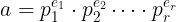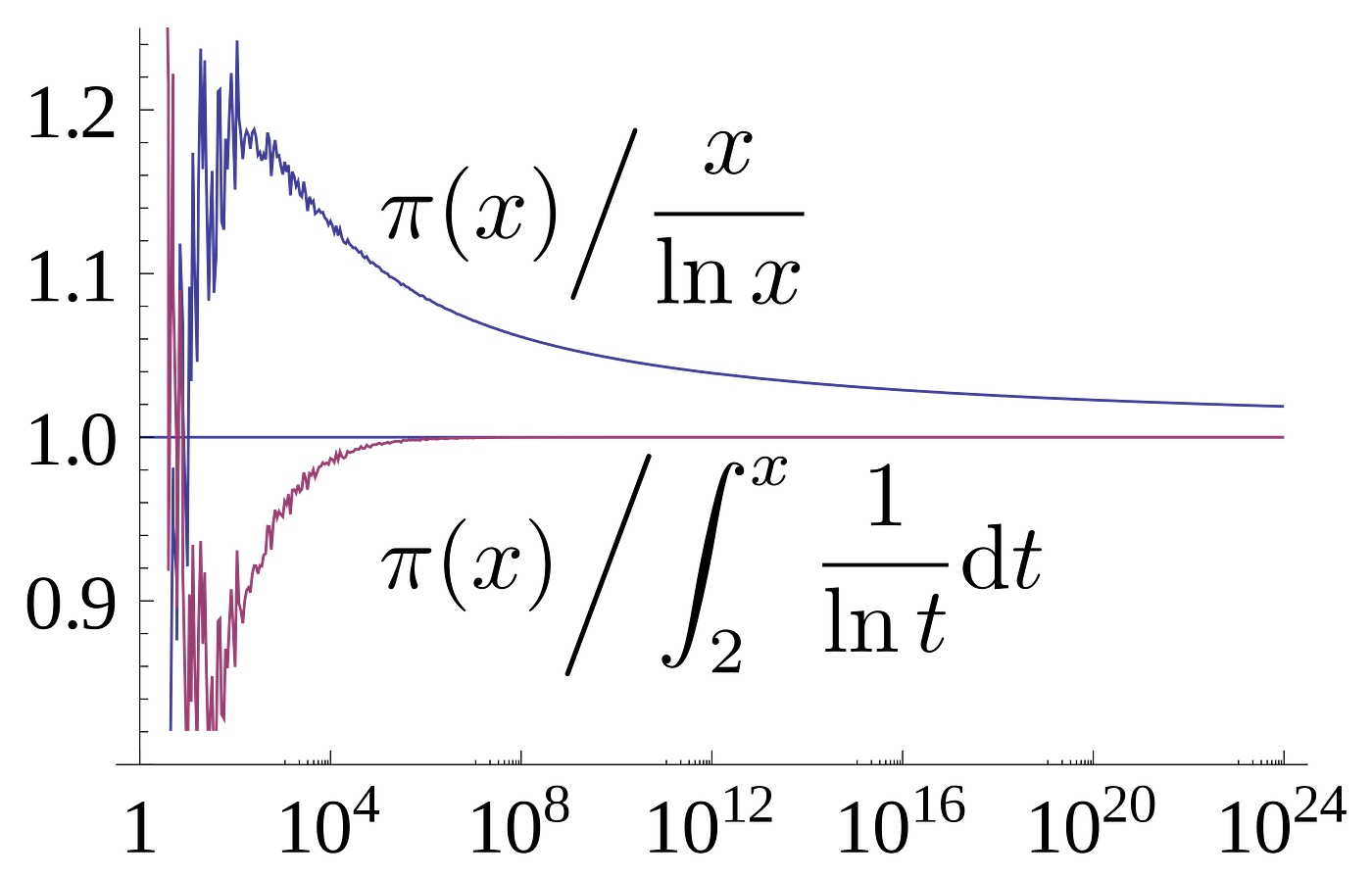# The building blocks of arithmetics

Prime numbers are considered the building blocks of arithmetics because no matter what natural number you may think of, you can express it as product of prime numbers. For instance, take 30, it can be decomposed as 2·3·5. Or 123456 in 2^6·3·643. The process of decomposing a number to its prime number factors is called it factorisation.unique factorisation of a composite number

# How many prime numbers are there? Can we find a “magic formula” to get them all?

It was proven by Euclid back in 300 BCE that there are infinitely many prime numbers. The largest one found as of August 2020 is 2^82589933-1 and has 24,862,048 digits when written in base 10.Ratio of prime number counting function to two approximations. From Wikipedia

# Primality testing and prime generation

In this section we investigate how to find prime numbers and test if an arbitrary number is prime.

## A naive way to generate primes

The natural way to generate prime numbers is to start from the smallest one {2} and test if the next one (3) is divisible by 2, since it is not, we add it to the list, then we have {2, 3}. We go to test 4 now, since it is divisible by one of the primes we already have (2) we don’t add it to the list and we try the next one, 5. 5 is not divisible neither by 2 nor by 3 so we add it to the list, {2, 3, 5} and so on… This is a very computationally intensive algorithm since all the time you have to check your full list that, by the way, is increasing every time you find a new prime.

## The sieve of Eratosthenes

A faster way to generate prime numbers is using the sieve of Eratosthenes where basically you build a list of all the natural numbers from 1 to n (n is the natural number below which you want all the primes) and remove all the multiples of the newly find prime. Say we want the prime numbers smaller than n=120. The algorithm starts with 2, then you discard its multiples (2, 4, 8, …, 120), you go for 3 and you know it is prime because it hasn’t been discarded, so you eliminate multiples of 3 that haven’t been discarded (3, 9, 12, 15…). The next number is 4 and has been discarded so you go to 5 and add it as prime, then eliminate its multiples… You can find a nice implementation in python here.

## Primality testing and Miller-Rabin algorithm

All the approaches so far seem to be very lengthly… in cryptography you often work with 256 bit prime numbers (2²⁵⁵ to 2²⁵⁶). Now, you can’t generate all prime numbers up to 2²⁵⁶ and then choose one at random, for this you need to use a test of primality. Basically what you do is draw a random natural number and then check whether this number is prime or not.